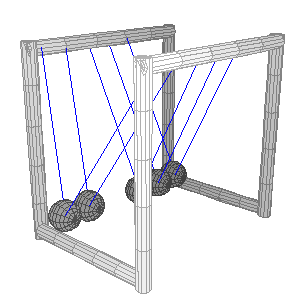#Difference Between Similar Terms and Objects

# Difference between force and momentumForce vs Momentum

Often the idea of force and momentum is confused with one another. Momentum is basically the amount of motion that is comprised within a moving object. Force, on the other hand, is the external action of pulling or pushing something. A force results in change of momentum.

Looking at this mathematically, force is mass times acceleration of an object whereas momentum is mass times acceleration of an object. The two quantities are linked through the following equation:

F=dP/dt;

that is force F, is the derivative with respect to time t, of momentum P.

The above mentioned link between force and momentum is given by newton’s second law, which states that the change of momentum of any object (which is force) is given by mass times acceleration.

Momentum changes if the velocity changes, whereas force changes when the acceleration changes. Force can remain constant even if there is a change in velocity, provided that the acceleration is constant.

Another link that can be noted between momentum and force through the above mentioned definitions is as follows:

momentum=mass x velocity
momentum= mass x (acceleration x time)
momentum=(mass x acceleration) x time
momentum=force x time

The key thing to note here is how momentum depends on time. Meaning the longer a force is applied, the amount of momentum increases. Contrarily, force does not depend on time in this manner. In fact, the increase in time actually decreases the amount force if the momentum is constant.

Force and momentum both are vector quantities. A vector quantity is something that has both magnitude and direction. The direction of momentum depends on the direction of velocity. The direction of force depends on acceleration.

Forces can also be balanced and unbalanced. A unbalanced force results in movement of an object. For a balanced force, the forces act in such a way that the magnitude is equal but the direction is opposite hence cancelling the net effect. For this reason an object does not move when forces are balanced and hence the velocity is zero, resulting in zero momentum. This means that forces can exist for a stationary object but the momentum is always zero for such an object.

Forces can be further classified into two types: contact forces and forces that act at a distance. Contact forces are those forces that are a result of actual touching between two objects, example: a ball hitting a bat. While, the second type of forces are those that act upon each other without any physical contact; such as gravitational force between the Earth and us. Momentum however has not been classified in such a way. Any unbalanced force acting upon a body will result in momentum.

All in all, the key thing to remember is that momentum is just a quantity that tell us about the content of motion present in a moving object, while force is a quantity that when acts upon an object changes this amount of motion.

Summary:

Momentum is amount of motion in a moving body, whereas force is an action of push or pull.
Force changes momentum of a body.
force=mass*acceleration; whereas momentum=mass*velocity
Force does not change for constant acceleration whereas momentum changes.
momentum and force are linked by F=dP/dt and momentum=force*time
Momentum increases with time for an applied force.
Momentum and force are both vector quantities.
The direction of momentum depends on the direction of velocity.
The direction of force depends on direction of acceleration.
Forces can be balanced and unbalanced.
For a stationary object forces need not be zero, but momentum is zero.
Forces can be classified into contact forces and those that act at a distance. Momentum cannot be classified in such a way.

`Image Credit  :http://commons.wikimedia.org/wiki/File:Newton_Cradle_5_ball_system_in_3D_2_ball_swing.gif`
Latest posts by marium (see all)

### Search DifferenceBetween.net :

Custom Search

Help us improve. Rate this post!(1 votes, average: 5.00 out of 5)Loading...Email This Post : If you like this article or our site. Please spread the word. Share it with your friends/family.

### 1 Comment

1. This is a good article but there is a funny mistake in the second paragraph:
“Looking at this mathematically, force is mass times acceleration of an object whereas momentum is mass times acceleration of an object.”
Of course you mean: “… whereas momentum is mass times VELOCITY of an object.”

Please note: comment moderation is enabled and may delay your comment. There is no need to resubmit your comment.

Articles on DifferenceBetween.net are general information, and are not intended to substitute for professional advice. The information is "AS IS", "WITH ALL FAULTS". User assumes all risk of use, damage, or injury. You agree that we have no liability for any damages.